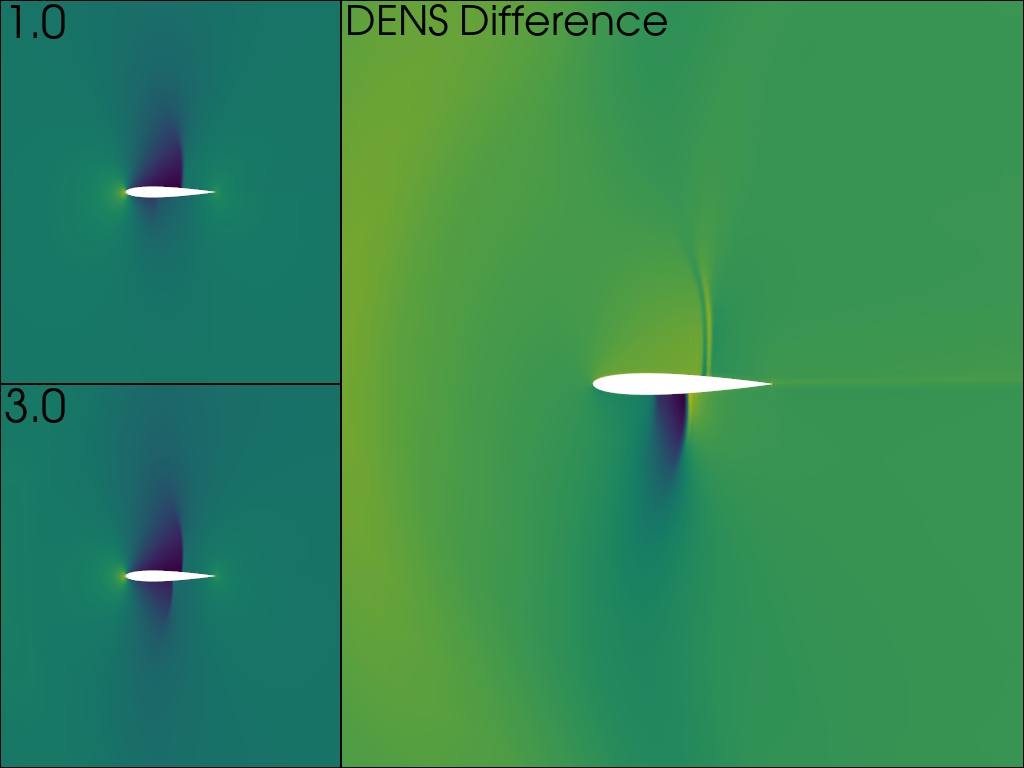To have more control over reading data files, use a class based reader. This class allows for more fine-grained control over reading datasets from files. See `pyvista.get_reader()` for a list of file types supported.

```import pyvista
from pyvista import examples
import numpy as np
from tempfile import NamedTemporaryFile
```

An XML PolyData file in `.vtp` format is created. It will be saved in a temporary file for this example.

```temp_file = NamedTemporaryFile('w', suffix=".vtp")
temp_file.name
```

Out:

```'/tmp/tmpqobcdt3c.vtp'
```

`pyvista.Sphere` already includes `Normals` point data. Additionally `height` point data and `id` cell data is added.

```mesh = pyvista.Sphere()
mesh['height'] = mesh.points[:, 1]
mesh['id'] = np.arange(mesh.n_cells)
mesh.save(temp_file.name)
```

`pyvista.read()` function reads all the data in the file. This provides a quick and easy one-liner to read data from files.

```new_mesh = pyvista.read(temp_file.name)
print(f"All arrays: {mesh.array_names}")
```

Out:

```All arrays: ['height', 'Normals', 'id']
```

Using `pyvista.get_reader()` enables more fine-grained control of reading data files. Reading in a `.vtp`` file uses the `pyvista.XMLPolyDataReader`.

```reader = pyvista.get_reader(temp_file.name)
```

Out:

```XMLPolyDataReader('/tmp/tmpqobcdt3c.vtp')
```

Some reader classes, including this one, offer the ability to inspect the data file before loading all the data. For example, we can access the number and names of point and cell arrays.

```print(f"Number of point arrays: {reader.number_point_arrays}")
```

Out:

```Number of point arrays: 2
Available point data:   ['Normals', 'height']
Number of cell arrays:  1
Available cell data:    ['id']
```

We can select which data to read by selectively disabling or enabling specific arrays or all arrays. Here we disable all the cell arrays and the `Normals` point array to leave only the `height` point array. The data is finally read into a pyvista object that only has the `height` point array.

```reader.disable_all_cell_arrays()
```

Out:

```Point array status: {'Normals': False, 'height': True}
Cell array status:  {'id': False}
```

We can reuse the reader object to choose different variables if needed.

```reader.enable_all_cell_arrays()
```

Out:

```New read arrays: ['height', 'id']
```

Some Readers support setting different time points or iterations. In both cases, this is done using the time point functionality. The NACA dataset has two such points with density. This dataset is in EnSight format, which uses the `pyvista.EnSightReader` class.

```filename = examples.download_naca(load=False)
print(f"Available time points: {time_values}")
```

Out:

```EnSightReader('/home/alex/.local/share/pyvista/examples/naca.bin.case')
Available time points: [1.0, 3.0]
Available point arrays: ['DENS']
```

First both time points are read in, and then the difference in density is calculated and saved on the second mesh. The read method of `pyvista.EnSightReader` returns a `pyvista.MultiBlock` instance. In this dataset, there are 3 blocks and the new scalar must be applied on each block.

```reader.set_active_time_value(time_values)

for block_0, block_1 in zip(mesh_0, mesh_1):
block_1['DENS_DIFF'] = block_1['DENS'] - block_0['DENS']
```

The value of DENS is plotted on the left column for both time points, and the difference on the right.

```plotter = pyvista.Plotter(shape='2|1')

plotter.subplot(0)

plotter.subplot(1)

# pyvista currently cannot plot the same mesh twice with different scalars
plotter.subplot(2)

plotter.camera_position= ((0.5, 0, 8), (0.5, 0, 0), (0, 1, 0))

plotter.show()
```Reading time points or iterations can also be utilized to make a movie. Compare to Create a GIF Movie, but here a set of files are read in through a ParaView Data format file. This file format and reader also return a `pyvista.MultiBlock` mesh.

```filename = examples.download_wavy(load=False)
```

Out:

```PVDReader('/home/alex/.local/share/pyvista/examples/wavy/wavy.pvd')
```

For each time point, plot the mesh colored by the height. Put iteration value in top left

```plotter = pyvista.Plotter(notebook=False, off_screen=True)
# Open a gif
plotter.open_gif("wave_pvd.gif")